Next: Paraxial Optics Up: Geometric Optics Previous: Example 12.1: The corner-cube

## Example 12,2: Refraction

Question: A light-ray of wavelength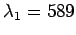nm traveling through air is incident on a smooth, flat slab of crown glass (refractive index 1.52) at an angle of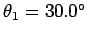to the normal. What is the angle of refraction? What is the wavelength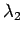of the light inside the glass? What is the frequencyof the light inside the glass?

Answer: Snell's law can be written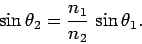In this case,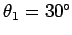,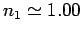(here, we neglect the slight deviation of the refractive index of air from that of a vacuum), and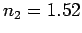. Thus,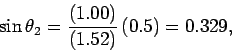giving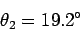as the angle of refraction (measured with respect to the normal).

The wavelengthof the light inside the glass is given by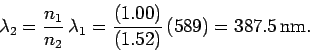The frequencyof the light inside the glass is exactly the same as the frequency outside the glass, and is given by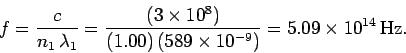Next: Paraxial Optics Up: Geometric Optics Previous: Example 12.1: The corner-cube
Richard Fitzpatrick 2007-07-14